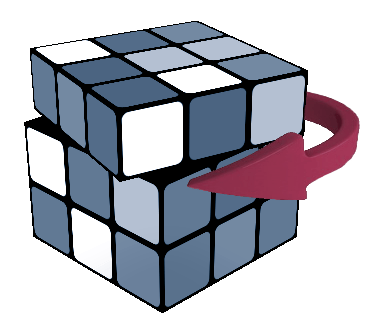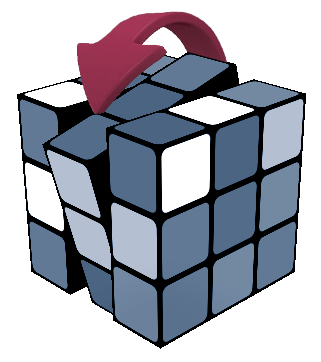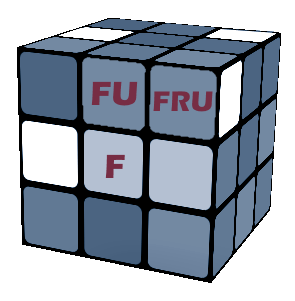Demonstrating face, slice, double and whole cube rotations. With a mark on the FRU corner.
Press Play to start.
On the Rubik's Cube Notation page I have presented how we mark the basic face, middle layer (slice) and cube rotations. Let's go further and discuss the advanced notation what you often meet while reading Rubik's Cube algorithms.

[hide]
[show]
Contents

## Face rotationsU: Up face clockwise
We mark the 6 faces of the Rubik's Cube with a letter according to our perspective as we hold the puzzle with one side facing us and an one parallel to the ground:
F (Front), U (Up), R (Right), B (Back), L (Left), D (Down).
F R U L B D

U - a 90-degree clockwise rotation of the upper face U
U' - a 90-degree counterclockwise rotation of the U face U'
U2 - a half turn of the upper face U2

## Slice turnsM: Middle layer like left face clockwise
Middle layer rotations or slice turns are not simply the rotations of two opposite layers because these moves reposition the centre cubelets too.
M - Middle layer turn - in the same direction as an L turn between R and L.
E - Equatorial layer - direction as a D turn between U and D.
S - Standing layer - direction as an F turn between F and B.
Note the following correlation:
M = L' R X'
M E S

## Two layers at the same timed or Dw: the two bottom layers clockwise
Double turns are marked with the lowercase letter of the corresponding face: f, u, r, b, l, d. For example d means the clockwise rotation of the two bottom layers. Dw means the same move but this notation is mainly used by Japanese cubers. Rarely they mark these turns with 2D (the number comes ahead because D2 means a double D).
Multiple-layer turns are necessary to solve higher order puzzles like the 4x4x4 Rubik's Revenge.
In some cases you might find Rubik's Cube algorithms with lowercase letters meaning the counterclockwise rotation of a face but this is rare.
d = D E

## Whole cube reorientationx: rotate the entire cube on R
The entire cube rotations are not necessary to solve the cube but we still use them in algorithms to reorient the puzzle. These moves can also be executed in the two directions and double turns are also possible.
x - rotate the entire cube on R (do an R move without holding the two other layers)
y - rotate the entire cube on U
z - rotate the entire cube on F

Whole cube rotations can be marked with either lower or uppercase letters.

## Big cube notationBig cubes allow deep turns and slices
The notation of the Rubik's Cube applies to the big cubes too but the deep turns and inner slice turns also come in.

2F - second inner front layer (inner slice)
F2 - the two outer front layers together (deep turn)
3Fw - The three front layers together on a big cube (min 7x7x7)
3Fw2 - 180 degree turn of the three front layers on a big cube
(in the case of the 4x4x4 cube we marked this with f and Fw )

## Piece notationFRU: front-right-up corner piece
There are three types of pieces on the Rubik's Cube: centre, edge and corner pieces.
There's only one centre piece belonging to every face so we mark these with the uppercase letter of the face it belongs to: F marks the front centre piece.
An edge piece is determined by the two faces it belongs to: FU - the front-up edge.
A corner piece is described by the three faces next to it: FRU front-right-up corner.

## Finger trick notations

You can find brackets in the Rubik's algorithms because we group together fragments (triggers) that are easy to execute.
There's no officially adopted finger trick notation but the DeeDubb (DW) notation for 3x3x3 cubes is widely used. This has been presented in the speedsolving.com forum.

Finger tricks are used in speedcubing to reduce the solution time. The goal is to describe a Rubiks' Cube algorithm in the most comfortable and efficient way to execute with the human hand. Taking down the hand off a side and repositioning always takes time so we're trying to execute more operations at the same time. The more overlapped moves and the less regrips, the faster you execute the operation. For this we use triggers which are short sequences of face turns which are easy and fast to execute. Usually you can see these fragments in brackets in the algorithms.

For example instead of doing R U F' we can simply do a R d R' avoiding the regrip.

This notation is based on describing three things: the finger, the puzzle piece and the grip of the fingers on the cube.

The fingers are marked with: T (thumb), I(index), M (middle), R(ring), P (pinkie), W (wrist move).

The piece notation is the same as described above on this page.

Grip notation describes how to hold the cube.
Ex. TF - right thumb on F, other fingers on the opposite side. TU - right thumb on up, the rest of the fingers on down.
The left thumb is marked with lowercase t.

An example: {M-R4, T-R3} R2 U S'(I2) U2' S(I1) U R2

Perform these moves on a 3D Rubik's Cube